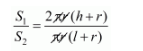# A right circular cone and a right circular cylinder have equal`
Question:

A right circular cone and a right circular cylinder have equal base and equal height. If the radius of the base and height are in the ratio 5 : 12, write the ratio of the total surface area of the cylinder to that of the cone.

Solution:

Given that

$r: h=5: 12$

i.e. $r=5 x, h=12 x$

Right, circular cone and right circular cylinder have equal base and equal right.

Therefore,

The total surface area of cylinder $S_{1}=2 \pi r(h+r)$

The total surface area of cone $S_{2}=2 \pi r(l+r)$

$l=\sqrt{r^{2}+h^{2}}$

$=\sqrt{25 x^{2}+144 x^{2}}$

$=\sqrt{169 x^{2}}$

$=\sqrt{169 x^{2}}$

Now,$=\frac{2(h+r)}{(I+r)}$

$\frac{S_{1}}{S_{2}}=\frac{2(12 x+5 x)}{13 x+5 x}$

$=\frac{2 \times 17 x}{18 x}$

$\frac{S_{1}}{S_{2}}=\frac{17}{9}$

Hence, $S_{1}: S_{2}: 17: 9$